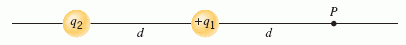# Magnitude of a Point Charge on a Line

theintarnets

## Homework Statement

The drawing shows a positive point charge +q1, a second point charge q2 that may be positive or negative, and a spot labeled P, all on the same straight line.
The distance d between the two charges is the same as the distance between q1 and the spot P.
With q2 present, the magnitude of the net electric field at P is twice what it is when q1 is present alone.
Given that q1 = +1.19 µC, determine the magnitude |q2| when q2 is positive. Determine the magnitude |q2| when q2 is negative.## Homework Equations

Actually my problem is I don't know which equation I'm supposed to use. All I know is that E = kq/r2

## The Attempt at a Solution

I tried making an equation, given that the net field at P is twice what q1 is alone. So I would then have something like:
2(kq/r2) = kqQ/2r2
I'm not sure if that's right though. I would just get 4 as an answer. And then I have no idea what to do about -q2...